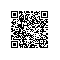# 比较全面的php session验证码与防识别

1、  背景干扰：干扰线、干扰点、干扰图，基本没有，程序很容易通过高亮度调节去除掉。

2、  字符旋转：破解机器人通过数次学习、旋转之后，能够得到 90% 以上的正确识别率，采用常规字体，能够得到接近 100% 的识别。

3、  随机间距：基本没用，采用提取高亮度之后，采用图片切割的方法，很容易就将随机间距消灭掉。

1、  背景干扰线尽量能够干扰到字符，采用和字符相同的颜色，能够破坏高亮度反差色提取法对字符的学习。 QQ有采用。

2、  矢量变形：想 Google 、 Ms 、 Yahoo 都采用了这种变态的方法，这种将字符进行扭曲变形，基本上机器识别率为零，因为没有相对应的固定形状。 损失是用户也不一定认得。需要验证码图片有一些大才行。

3、  字符粘连，可以破坏掉字符切割法分割字符， Google 也有用到这个， QQ 也有采用。

4、  中文验证码，中文验证码的识别难度比较大，但是现在逐渐的也慢慢被学习并且破解。（香港，台湾是用繁体）

5、  字符旋转：需要和字符粘连在一起才能够起作用，他们一起，验证码几乎不具备机器破解可能性。 加随机背景图片、随机字体、稍微旋转字母或数字，位置不固定，稍微扭曲

8.         case '1' :
9.             $str = '123456789'; 10. break; 11. case '2' : 12.$str = 'abcdefghijklmnopqrstuvwxyz';
13.             break;
14.         case '3' :
15.             $str = 'ABCDEFGHIJKLMNOPQRSTUVWXYZ'; 16. break; 17. case '4' : 18.$str = 'ABCDEFGHIJKLMNOPQRSTUVWXYZabcdefghijklmnopqrstuvwxyz';
19.             break;
20.         case '5' :
21.             $str = 'ABCDEFGHIJKLMNPQRSTUVWXYZ123456789'; 22. break; 23. case '6' : 24.$str = 'abcdefghijklmnopqrstuvwxyz1234567890';
25.             break;
26.         case '7' ://中文验证码
27.             break;
28.         default :
29.             $str = 'ABCDEFGHIJKLMNPQRSTUVWXYZabcdefghijkmnpqrstuvwxyz23456789'; 30. break; 31. } 32.$result = '';
33.
34.     for($i = 0;$i < $length;$i ++) {
35.         if ($mode == 7) { 36.$str [$i] = chr ( mt_rand ( 176215 ) ) . chr ( mt_rand ( 161249 ) ); 37.$str [$i] = iconv ( "GB2312""UTF-8",$str [$i] ); //imagettftext是utf-8的,所以先转换下 38.$result .= $str [$i];
39.         } else {
40.             $l = strlen ($str ) - 1;
41.             $num = mt_rand ( 0,$l );
42.             $result .=$str [$num]; 43. } 44. } 45. return$result;
46. }
47.
48. //建立验证图片
49. function createAuthNumImg($randStr,$fontName, $imgW = 100,$imgH = 40) {
50.     header ( "content-type: image/png" );
51.     $image = imagecreate ($imgW, $imgH ); 52.$fontSize = 20;//字号
53.     //$green = imagecolorallocate($image,0x6b,0xc1,0x46);
54.     $gray = imagecolorallocate ($image, 228228228 ); //灰色
55.     $red = imagecolorallocate ($image, 255102204 );//粉色
56.     $blue = imagecolorallocate($image,0x53,0x68,0xbd);
57.     $colors = array($red, $gray,$blue);
58.
59.     $color_b = imagecolorallocate ($image, 000 ); //黑色
60.     for($i = 0;$i < 1000; $i ++) { //绘背景干扰点 61. imagesetpixel ($image, mt_rand ( 0, $imgW ), mt_rand ( 0,$imgH ), $colors[rand(0,count($colors)-1)]);
62.     }
63.     imagerectangle ( $image, 00,$imgW - 1, $imgH - 1,$color_b );//绘制边框
64.     imagettftext ( $image,$fontSize, 5325, $color_b,$fontName, $randStr);///将验证字符绘入图片 字符旋转 65. for($i=0; $i<2;$i++){ //绘背景干扰线
66.         imageline($image, mt_rand(0,5), mt_rand(6,18), mt_rand(65,$imgW), mt_rand(6,$imgH),$color_b);//一条干扰线
67.     }
68.     imagepng ( $image ); 69. imagedestroy ($image );
70. }
71.
72. session_start ();
73. $verifyCode = GetCode ( 5 ); 74.$_SESSION ['VERIFY_CODE'] = $verifyCode ; 75. createAuthNumImg ($verifyCode, "font.ttf"7530); //字体存放路径,如果你没有文件就去C:\WINDOWS\Fonts文件中找一个吧。
76.
77. /** 问答模式
78. $a=GetCode(2,1); 79.$b=GetCode(1,1);
80. $passPort =$a."+".$b."=?"; 81.$verifyCode = $a+$b;
82.
83. $_SESSION ['VERIFY_CODE'] =$verifyCode ;
84. createAuthNumImg ( \$passPort, "font.ttf", 75, 30);
85. */
86. ?>

1. <?php echo strlen('中'); echo strlen('ad');?>

1. <img src="validimg.jsp" alt="看不清？请刷新" width="50" height="20" onclick="this.src=this.src+'?'+Math.random()" />

1. <img src="validimg.jsp" width="50" height="20" > <a href="JavaScript:reloadImage("validimg.jsp" );">刷新</a>
2. <script language="JavaScript">
4. document.formName.img1.src = url+Math.random();
5. }
6. </script>使用钉钉扫一扫加入圈子
+ 订阅